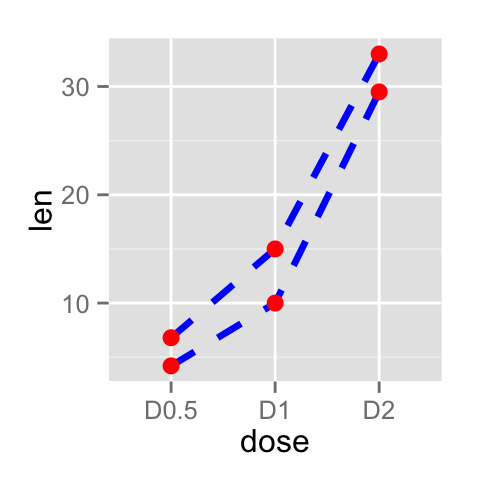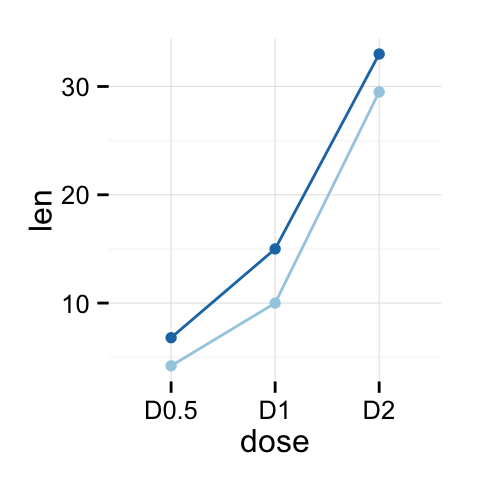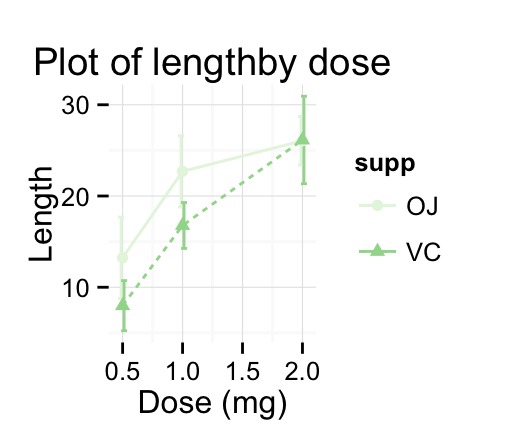ggplot2 line plot : Quick start guide - R software and data visualization

This R tutorial describes how to create line plots using R software and ggplot2 package.

In a line graph, observations are ordered by x value and connected.

The functions geom_line(), geom_step(), or geom_path() can be used.

x value (for x axis) can be :

• date : for a time series data
• texts
• discrete numeric values
• continuous numeric valuesBasic line plots

Data

Data derived from ToothGrowth data sets are used. ToothGrowth describes the effect of Vitamin C on tooth growth in Guinea pigs.

df <- data.frame(dose=c("D0.5", "D1", "D2"),
len=c(4.2, 10, 29.5))
##   dose  len
## 1 D0.5  4.2
## 2   D1 10.0
## 3   D2 29.5
• len : Tooth length
• dose : Dose in milligrams (0.5, 1, 2)

Create line plots with points

library(ggplot2)
# Basic line plot with points
ggplot(data=df, aes(x=dose, y=len, group=1)) +
geom_line()+
geom_point()
# Change the line type
ggplot(data=df, aes(x=dose, y=len, group=1)) +
geom_line(linetype = "dashed")+
geom_point()
# Change the color
ggplot(data=df, aes(x=dose, y=len, group=1)) +
geom_line(color="red")+
geom_point()Read more on line types : ggplot2 line types

You can add an arrow to the line using the grid package :

library(grid)
ggplot(data=df, aes(x=dose, y=len, group=1)) +
geom_line(arrow = arrow())+
geom_point()
# Add a closed arrow to the end of the line
myarrow=arrow(angle = 15, ends = "both", type = "closed")
ggplot(data=df, aes(x=dose, y=len, group=1)) +
geom_line(arrow=myarrow)+
geom_point()Observations can be also connected using the functions geom_step() or geom_path() :

ggplot(data=df, aes(x=dose, y=len, group=1)) +
geom_step()+
geom_point()
ggplot(data=df, aes(x=dose, y=len, group=1)) +
geom_path()+
geom_point()• geom_line : Connecting observations, ordered by x value
• geom_path() : Observations are connected in original order
• geom_step : Connecting observations by stairs

Line plot with multiple groups

Data

Data derived from ToothGrowth data sets are used. ToothGrowth describes the effect of Vitamin C on tooth growth in Guinea pigs. Three dose levels of Vitamin C (0.5, 1, and 2 mg) with each of two delivery methods [orange juice (OJ) or ascorbic acid (VC)] are used :

df2 <- data.frame(supp=rep(c("VC", "OJ"), each=3),
dose=rep(c("D0.5", "D1", "D2"),2),
len=c(6.8, 15, 33, 4.2, 10, 29.5))
##   supp dose  len
## 1   VC D0.5  6.8
## 2   VC   D1 15.0
## 3   VC   D2 33.0
## 4   OJ D0.5  4.2
## 5   OJ   D1 10.0
## 6   OJ   D2 29.5
• len : Tooth length
• dose : Dose in milligrams (0.5, 1, 2)
• supp : Supplement type (VC or OJ)

Create line plots

In the graphs below, line types, colors and sizes are the same for the two groups :

# Line plot with multiple groups
ggplot(data=df2, aes(x=dose, y=len, group=supp)) +
geom_line()+
geom_point()
# Change line types
ggplot(data=df2, aes(x=dose, y=len, group=supp)) +
geom_line(linetype="dashed", color="blue", size=1.2)+
geom_point(color="red", size=3)Change line types by groups

In the graphs below, line types and point shapes are controlled automatically by the levels of the variable supp :

# Change line types by groups (supp)
ggplot(df2, aes(x=dose, y=len, group=supp)) +
geom_line(aes(linetype=supp))+
geom_point()
# Change line types and point shapes
ggplot(df2, aes(x=dose, y=len, group=supp)) +
geom_line(aes(linetype=supp))+
geom_point(aes(shape=supp))It is also possible to change manually the line types using the function scale_linetype_manual().

# Set line types manually
ggplot(df2, aes(x=dose, y=len, group=supp)) +
geom_line(aes(linetype=supp))+
geom_point()+
scale_linetype_manual(values=c("twodash", "dotted"))You can read more on line types here : ggplot2 line types

Change line colors by groups

Line colors are controlled automatically by the levels of the variable supp :

p<-ggplot(df2, aes(x=dose, y=len, group=supp)) +
geom_line(aes(color=supp))+
geom_point(aes(color=supp))
pIt is also possible to change manually line colors using the functions :

• scale_color_manual() : to use custom colors
• scale_color_brewer() : to use color palettes from RColorBrewer package
• scale_color_grey() : to use grey color palettes
# Use custom color palettes
p+scale_color_manual(values=c("#999999", "#E69F00", "#56B4E9"))
# Use brewer color palettes
p+scale_color_brewer(palette="Dark2")
# Use grey scale
p + scale_color_grey() + theme_classic()Read more on ggplot2 colors here : ggplot2 colors

Change the legend position

p <- p + scale_color_brewer(palette="Paired")+
theme_minimal()
p + theme(legend.position="top")
p + theme(legend.position="bottom")
# Remove legend
p + theme(legend.position="none")The allowed values for the arguments legend.position are : “left”,“top”, “right”, “bottom”.

Read more on ggplot legend : ggplot2 legend

Line plot with a numeric x-axis

If the variable on x-axis is numeric, it can be useful to treat it as a continuous or a factor variable depending on what you want to do :

# Create some data
df2 <- data.frame(supp=rep(c("VC", "OJ"), each=3),
dose=rep(c("0.5", "1", "2"),2),
len=c(6.8, 15, 33, 4.2, 10, 29.5))
##   supp dose  len
## 1   VC  0.5  6.8
## 2   VC    1 15.0
## 3   VC    2 33.0
## 4   OJ  0.5  4.2
## 5   OJ    1 10.0
## 6   OJ    2 29.5
# x axis treated as continuous variable
df2\$dose <- as.numeric(as.vector(df2\$dose))
ggplot(data=df2, aes(x=dose, y=len, group=supp, color=supp)) +
geom_line() + geom_point()+
scale_color_brewer(palette="Paired")+
theme_minimal()
# Axis treated as discrete variable
df2\$dose<-as.factor(df2\$dose)
ggplot(data=df2, aes(x=dose, y=len, group=supp, color=supp)) +
geom_line() + geom_point()+
scale_color_brewer(palette="Paired")+
theme_minimal()Line plot with dates on x-axis

economics time series data sets are used :

##         date   pce    pop psavert uempmed unemploy
## 1 1967-06-30 507.8 198712     9.8     4.5     2944
## 2 1967-07-31 510.9 198911     9.8     4.7     2945
## 3 1967-08-31 516.7 199113     9.0     4.6     2958
## 4 1967-09-30 513.3 199311     9.8     4.9     3143
## 5 1967-10-31 518.5 199498     9.7     4.7     3066
## 6 1967-11-30 526.2 199657     9.4     4.8     3018

Plots :

# Basic line plot
ggplot(data=economics, aes(x=date, y=pop))+
geom_line()
# Plot a subset of the data
ggplot(data=subset(economics, date > as.Date("2006-1-1")),
aes(x=date, y=pop))+geom_line()Change line size :

# Change line size
ggplot(data=economics, aes(x=date, y=pop, size=unemploy/pop))+
geom_line()Line graph with error bars

The function below will be used to calculate the mean and the standard deviation, for the variable of interest, in each group :

#+++++++++++++++++++++++++
# Function to calculate the mean and the standard deviation
# for each group
#+++++++++++++++++++++++++
# data : a data frame
# varname : the name of a column containing the variable
#to be summariezed
# groupnames : vector of column names to be used as
# grouping variables
data_summary <- function(data, varname, groupnames){
require(plyr)
summary_func <- function(x, col){
c(mean = mean(x[[col]], na.rm=TRUE),
sd = sd(x[[col]], na.rm=TRUE))
}
data_sum<-ddply(data, groupnames, .fun=summary_func,
varname)
data_sum <- rename(data_sum, c("mean" = varname))
return(data_sum)
}

Summarize the data :

df3 <- data_summary(ToothGrowth, varname="len",
groupnames=c("supp", "dose"))
##   supp dose   len       sd
## 1   OJ  0.5 13.23 4.459709
## 2   OJ  1.0 22.70 3.910953
## 3   OJ  2.0 26.06 2.655058
## 4   VC  0.5  7.98 2.746634
## 5   VC  1.0 16.77 2.515309
## 6   VC  2.0 26.14 4.797731

The function geom_errorbar() can be used to produce a line graph with error bars :

# Standard deviation of the mean
ggplot(df3, aes(x=dose, y=len, group=supp, color=supp)) +
geom_errorbar(aes(ymin=len-sd, ymax=len+sd), width=.1) +
geom_line() + geom_point()+
scale_color_brewer(palette="Paired")+theme_minimal()
# Use position_dodge to move overlapped errorbars horizontally
ggplot(df3, aes(x=dose, y=len, group=supp, color=supp)) +
geom_errorbar(aes(ymin=len-sd, ymax=len+sd), width=.1,
position=position_dodge(0.05)) +
geom_line() + geom_point()+
scale_color_brewer(palette="Paired")+theme_minimal()Customized line graphs

# Simple line plot
# Change point shapes and line types by groups
ggplot(df3, aes(x=dose, y=len, group = supp, shape=supp, linetype=supp))+
geom_errorbar(aes(ymin=len-sd, ymax=len+sd), width=.1,
position=position_dodge(0.05)) +
geom_line() +
geom_point()+
labs(title="Plot of lengthby dose",x="Dose (mg)", y = "Length")+
theme_classic()
# Change color by groups
p <- ggplot(df3, aes(x=dose, y=len, group = supp, color=supp))+
geom_errorbar(aes(ymin=len-sd, ymax=len+sd), width=.1,
position=position_dodge(0.05)) +
geom_line(aes(linetype=supp)) +
geom_point(aes(shape=supp))+
labs(title="Plot of lengthby dose",x="Dose (mg)", y = "Length")+
theme_classic()
p + theme_classic() + scale_color_manual(values=c('#999999','#E69F00'))Change colors manually :

p + scale_color_brewer(palette="Paired") + theme_minimal()
# Greens
p + scale_color_brewer(palette="Greens") + theme_minimal()
# Reds
p + scale_color_brewer(palette="Reds") + theme_minimal()Infos

This analysis has been performed using R software (ver. 3.1.2) and ggplot2 (ver. 1.0.0)